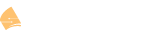# EIGRP K Values Formula

(Rene Molenaar) #1

This topic is to discuss the following lesson:

Eigrp Metric
(Rene Molenaar) #2

Hello Rene,

this was a really good post! One statement that I personally dont liked:

“ou don’t want your EIGRP routers calculating 24/7 and sending updates to each other just because the load or reliability of an interface has changed. We want routing protocols to be nice and quiet and only base their routing decisions on static values like bandwidth and delay.”

I think everyone would love to have a routing protocol that dynamically adapts to the network state but the processing overhead for the routers in your network would be a nightmare.

Thank you for this post.

Marek

(system) #3

Hi Rene,

AFAIK the formula when considered only BW and delay should be:

Metric= [ (10^7/ Minimum BW) + (sum of delay/10) ] * 256

(system) #4

Hello Rene,
Excellent explanation on the metric values used by EIGRP to determine “best” path (route) to take when trying to reach another non-local network. I always was curious how 'best" path was determine by EIGRP when deciding which way to send the packet when multiple paths exists, excellent. Of course this has no real bearing on “stub” networks I take.

Thanks again for your concise explanations on Networking w/ Cisco

Darren

(Rene Molenaar) #5

Hi Darren,

You are welcome. If you want to take a closer look at this then I can recommend to boot up some routers and look at the EIGRP topology table. Spend some time looking at the successor and feasible successor routers and check why it installed certain things in the routing table.

Rene

(system) #6

sorry i figured it out, myself, smaller metric is better, its all for influencing, i understand that now, thanks

(system) #7

EIGRP Metric = 256*[(K1 x BW) + ((K2 x BW)/(256-Load)) + (K3 x Delay) x ((K5/(Reliability + K4))]

Known as EIGRP “Distance” on the exam.

(Hussein Samir) #8

Hi Rene,

What is the effect of MTU (K5) value to the total metrics ? I mean the metric will be better if the MTU value was high or vice versa ?

(Hussein Samir) #9

I notice that you said “the MTU is being exchanged between EIGRP neighbors but not used for the metric calculation” so what is the benefit from this exchanging since it is not used for metric calculation ?

(Hussein Samir) #10

Hi Rene,

I understood that if the delay was in ten of microsecond we only sum the delays of the links and if the delay was in microsecond we need to divide the sum on 10 and put the value to formula, and about BW I do not understand why we multiply by 10^7 when I read in Wiki I understand the we do that to make the formula weighted and make measuring units harmonized but I do not understand how ? I mean why 10^7 ? from where this number come ?

(Hussein Samir) #11

Hi Rene,

Is there a formula to calculate the the AD ( Advertised Distance ) ?

(Hussein Samir) #12

Hi Rene,

What is the threshold value of FD ( the metric value ) that indicates to infinity ?

thanks.

(Rene Molenaar) #13

EIGRP uses a 32 bit metric so the value used for infinity is 4294967295, the highest value you can create.

(Rene Molenaar) #14

Hi Hussein,

The MTU doesn’t influence the metric, it’s not used in the metric calculation.

Rene

(Rene Molenaar) #15

Apparently when you have two equal cost paths and EIGRP has to select one of them, it will use the path with the highest MTU. The low MTU path will not be used.

(Rene Molenaar) #16

Hi Hussein,

Good question, I tried to look it up but I couldn’t find anything why they picked 10^7…can’t find any explanation.

Rene

(Rene Molenaar) #17

Hi Hussein,

The advertised distance that a router advertises to a neighbor is the feasible distance that it has stored for a route, it’s the same calculation.

Rene

(Diego M) #18

Hi Rene,

Here you see the result of changing the bandwidth on the interface. Something to remember is that EIGRP will use the highest bandwidth in the path from A to B (since this is the bottleneck).
Is this right?. A think that EIGRP use the lowest bandwitch.

Thanks you

(Rene Molenaar) #19

Hi Diego,

That is correct, I just fixed it. Thanks for reporting!

Rene

(Efrain V) #20

I’m about 2 years too late but I’d like to take a crack at this for future reference. The reason 10^7 is used is because 10^7=10,000,000 kbps which is the same things as 10Gbps. When this formula was developed into what it is today, 10gbps was insanely, insanely fast and was decided to be used as the number utilized to calculate the weight of the minimum bandwidth number in the EIGRP formula.

The reason that we are adding weight to each number in EIGRP is easy to understand. EIGRP is a complex formula with different sets of numbers that have varying levels of relevance. We decide how much weight, or relevance, the minimum bandwidth has by taking 10^7 (dividend) and dividing it by the minimum bandwidth (divisor), the resulting number (quotient) multiplied by 256 [Read: 2^8 ‘binary octet max value’] is your weighted bandwidth.

“The multiplication of 256 is done so EIGRP is compatible with IGRP (the predecessor of EIGRP).”